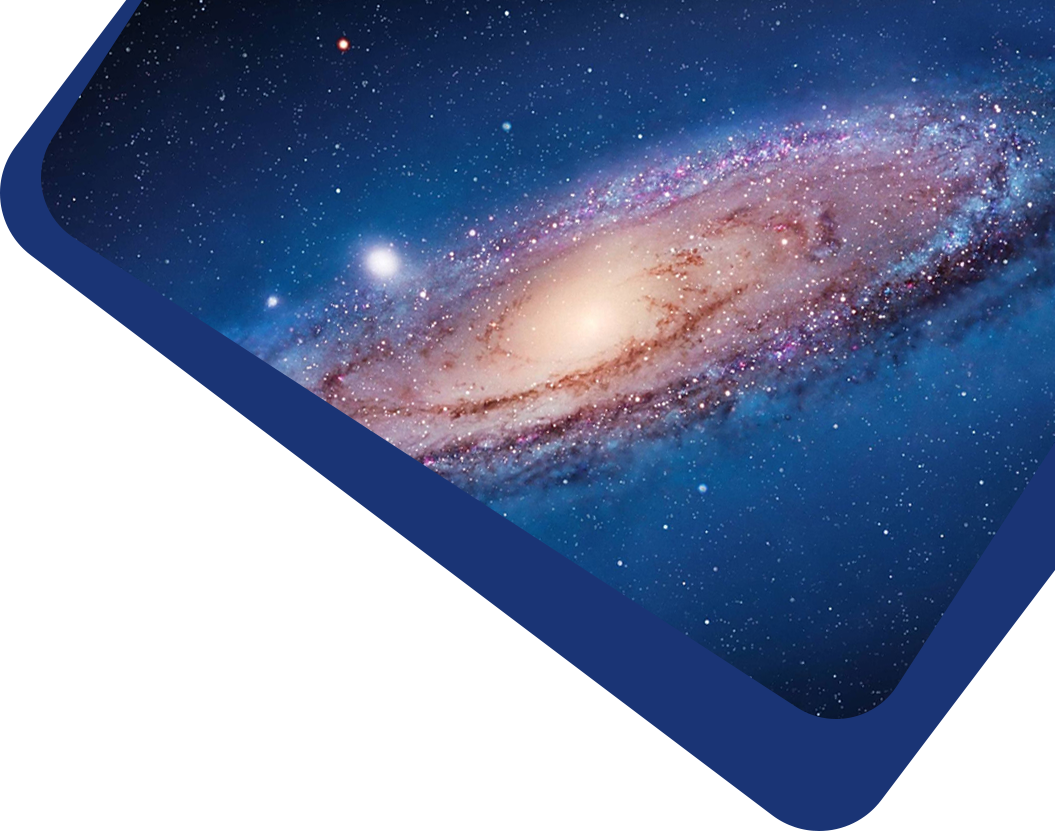Vol 19, No 4

## Field theory of the correlation function of mass density fluctuations for self-gravitating systems

#### Yang Zhang, Qing Chen, Shu-Guang Wu

Abstract

Abstract The mass density distribution of Newtonian self-gravitating systems is studied analytically in the field theoretical method. Modeling the system as a fluid in hydrostatic equilibrium, we apply Schwinger’s functional derivative on the average of the field equation of mass density, and obtain the field equation of 2-point correlation function ξ(r) of the mass density fluctuation, which includes the next order of nonlinearity beyond the Gaussian approximation. The 3-point correlation occurs hierarchically in the equation, and is cut off by the Groth-Peebles ansatz, making it closed. We perform renormalization and write the equation with three nonlinear coefficients. The equation tells us that ξ depends on the point mass m and the Jeans wavelength scale λ0, which are different for galaxies and clusters. Applying this to large scale structures, it predicts that the profile of ξcc for clusters is similar to ξgg for galaxies but with a higher amplitude, and that the correlation length increases with the mean separation between clusters, i.e., a scaling behavior r0 ≃ 0.4d. The solution yields the galaxy correlation ξgg (r) ≃ (r0/r)1.7 valid only in a range 1 < r < 10 h−1 Mpc. At larger scales the solution ξgg deviates below the power law and goes to zero around ∼50 h−1 Mpc, just as the observations show. We also derive the field equation of the 3-point correlation function in the Gaussian approximation and its analytical solution, for which the Groth-Peebles ansatz with Q = 1 holds.

Keywords

Keywords cosmology: theory — cosmology: large-scale structure of universe

Full Text
Refbacks

• There are currently no refbacks.